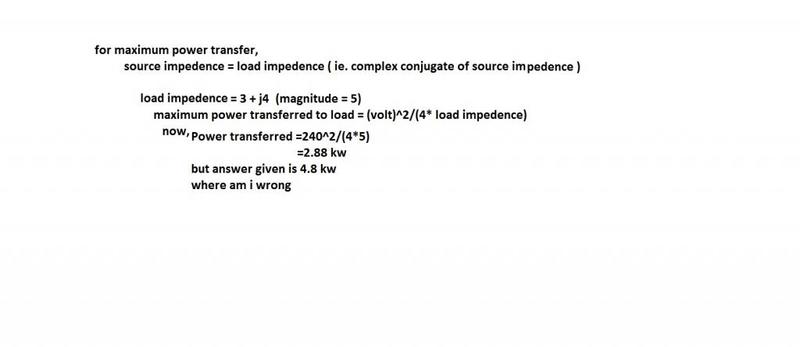# Maximum power transfer

1. Homework Statement
A voltage source of 240 v having an internal impedance of (3-j4) ohm, is supplying power to a complex load impedance Z1. What will be the maximum power transferred to load?

2. Homework Equations

3. The Attempt at a Solution## Answers and Replies

gneill
Mentor
You want to look at the real power delivered to the load. So you don't want to incorporate the imaginary parts of the impedances and use the magnitude.

One quick & dirty shortcut here is that since the two impedances are in series and are complex conjugates, their sum is purely real and comprised of two equal pieces; only the two real components are "seen" by the source since the complex bits cancel. That makes it dead easy to calculate the current and voltage across the load...

rude man
Homework Helper
Gold Member
Since you haven't defined the load impedance numerically, the problem has no solution.

If the load impedance is the complex conjugate of the source impedance then gneill has given you a good hint.

NascentOxygen
Staff Emeritus
Science Advisor
A voltage source of 240 v having an internal impedance of (3-j4) ohm, is supplying power to a complex load impedance Z1. What will be the maximum power transferred to load?
With the load connected, what is the total circuit impedance?

With the load connected, what will be the magnitude of the current?

NascentOxygen
Staff Emeritus
Science Advisor
Since you haven't defined the load impedance numerically,
It is not necessary to do so. We are looking for the maximum maximum power.

rude man
Homework Helper
Gold Member
It is not necessary to do so. We are looking for the maximum maximum power.

OK. I did not see the horizontal scroll bar so the word 'maximum' was hidden.